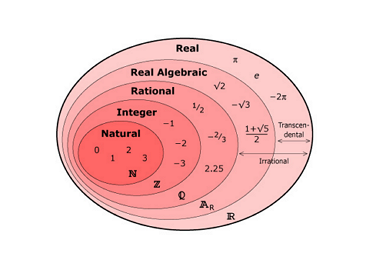# The ol' switcheroo function

Calculus Level 3True or False:

There exists a continuous function $f:\mathbb{R}\to\mathbb{R}$ such that $f(x)$ is rational whenever $x$ is irrational and irrational whenever $x$ is rational.

×